# Engineering Mechanics - KOP: Impulse and Momentum

### Exercise :: KOP: Impulse and Momentum - General Questions

• KOP: Impulse and Momentum - General Questions
11.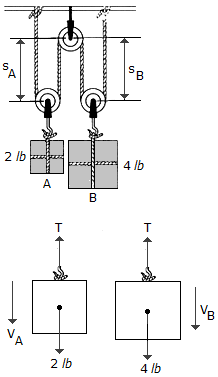Determine the velocities of blocks A and B 2 s after they are released from rest. Neglect the mass of the pulleys and cables.

 A. vA = 21.5 ft/s 8, VB = 21.5 ft/s 9 B. vA = 64.4 ft/s 8, VB = 64.4 ft/s 9 C. vA = 64.4 ft/s 8, VB = 32.2 ft/s 9 D. vA = 21.5 ft/s 8, VB = 10.73 ft/s 9

Explanation:

No answer description available for this question. Let us discuss.

12.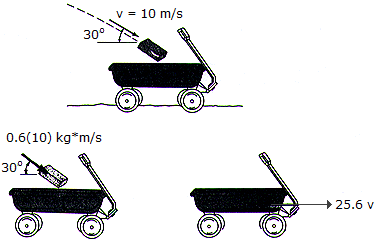A 0.6-kg brick is thrown into a 25-kg wagon which is initially at rest. If, upon entering, the brick has a velocity of 10 m/s as shown, determine the final velocity of the wagon.

 A. vwagon = 0.203 m/s B. vwagon = 0.208 m/s C. vwagon = 0.240 m/s D. vwagon = 0.234 m/s

Explanation:

No answer description available for this question. Let us discuss.

13.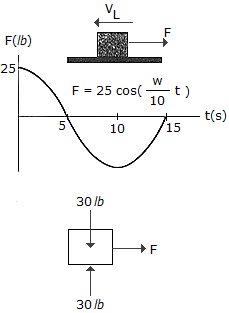A 30-lb block is initially moving along a smooth horizontal surface with a speed of v1 = 6 ft/s to the left. If it is acted upon by a force F, which varies in the manner shown, determine the velocity of the block in 15 s. The argument for the cosine is in radians.

 A. v2 = 91.4 ft/s 7 B. v2 = 79.4 ft/s 6 C. v2 = 91.4 ft/s 6 D. v2 = 79.4 ft/s 7

Explanation:

No answer description available for this question. Let us discuss.

14.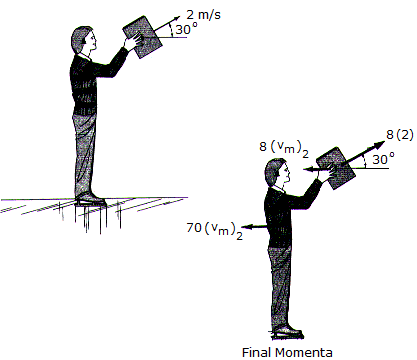A man wearing ice skates throws an 8-kg block with an initial velocity of 2 m/s, measured relative to himself, in the direction shown. If he is originally at rest and completes the throw in 1.5 s while keeping his legs rigid, determine the horizontal velocity of the man just after releasing the block. What is the average vertical reaction of both his skates on the ice during the throw? The man has a mass of 70 kg. Neglect friction and the motion of his arms.

 A. vman = 0.1776 m/s, Navg = 765 N B. vman = 0.1979 m/s, Navg = 771 N C. vman = 0.1776 m/s, Navg = 771 N D. vman = 0.1979 m/s, Navg = 765 N

Explanation:

No answer description available for this question. Let us discuss.

15.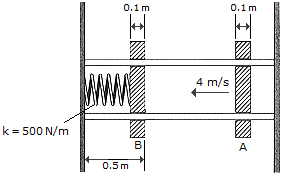Plates A and B each have a mass of 4 kg and are restricted to move along the frictionless guides. If the coefficient of restitution between the plates is e = 0.7, determine (a) the speed of both plates just after collision and (b) the maximum deflection of the spring. Plate A has a velocity of 4 m/s just before striking B. Plate B is originally at rest.

 A. vA2 = 0.857 m/s, vB2 = 4.86 m/s, x = 0.434 m B. vA2 = 0.600 m/s, vB2 = 3.40 m/s, x = 0.304 m C. vA2 = 0, vB2 = 4.00 m/s, x = 0.358 m D. vA2 = 0.327 m/s, vB2 = 3.67 m/s, x = 0.329 m